# Calibration of Regression Diagnostics

Indications of departures from regression assumptions in diagnostic plots may reflect sampling variation. This is an especial issue for relatively small datasets. Diagnostic plots for a number of sets of simulated data may be an essential aid to judgement. In effect, the observed diagnostic plot is judged against a simulated sampling distribution for such plots.

## A ‘simple’ straight line regression example

We use data, from the DAAG PACKAGE, that compares record times for Northern Island hill races between males and females:

library(DAAG, warn.conflicts=FALSE)
library(latticeExtra)
#> Loading required package: lattice

The data that are plotted in Figure 1 are, as they stand, problematic for least squares fitting. A least squares line has nevertheless been added to the plot. The discussion that immediately follows highlights problems that would largely be avoided if a logarithmic transformation had first been applied on both axes.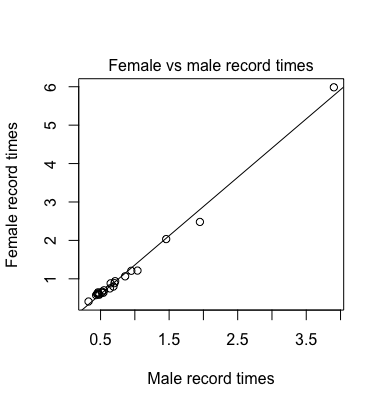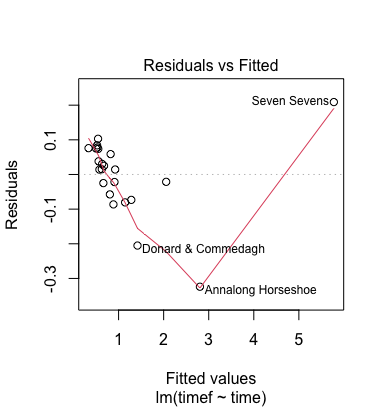Figure 1: Record times for hill races are compared – females versus males.

Code is:

plot(timef~time, data=nihills,
xlab="Male record times",
ylab="Female record times")
mtext("Female vs male record times", side=3, line=0.25)
mftime.lm <- lm(timef ~ time, data=nihills)
abline(mftime.lm)
plot(mftime.lm, which=1)

Figure 2 shows the four default diagnostic plots from the regression on timef on time.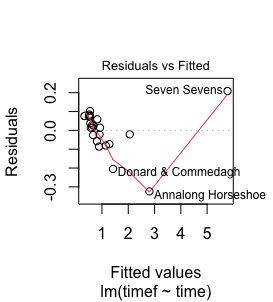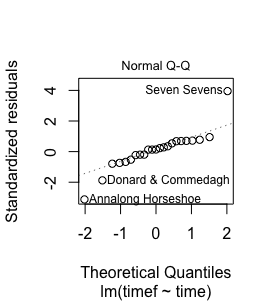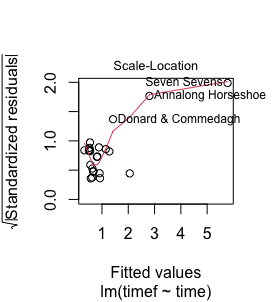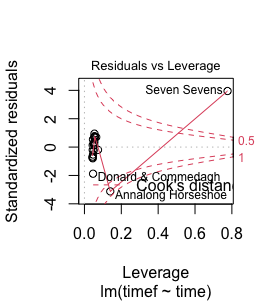Figure 2: Diagnostic plots from the regession of timef on time.

## The function plotSimScat()

The function plotSimScat() is designed for use with straight line regression. It plots either actual data values and simulated values against the $$x$$-variable, or residuals and simulated residuals.

Figure 3 shows four scatterplots that overlay residuals from the actual data with residuals that are simulated from the model. The coefficients used are those for the fitted least squares line, and the standard deviation is the estimate that is returned by R’s lm() function.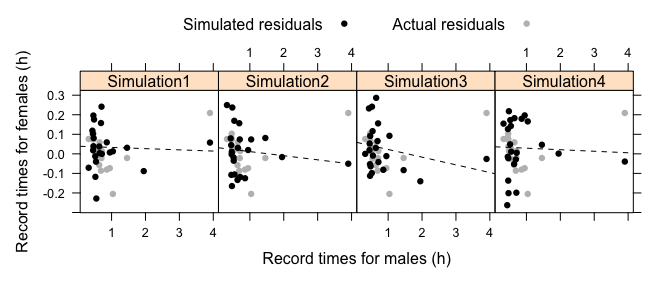Figure 3: The plots are four simulations of points. The coefficients used, and the standard deviation, are from the fitted least squares line. The residuals from the fitted model are in gray.

The largest simulated value lies consistently above the data value. Code is:

mftime.lm <- lm(timef ~ time, data=nihills)
gph <- plotSimScat(mftime.lm, show="residuals")
gph <- update(gph, xlab="Record times for males (h)",
ylab="Record times for females (h)")
print(gph)

# Diagnostic Plots for Simulated Data – plotSimDiags()

The function plotSimDiags() can be used with any lm object, or object of a class that inherits from lm. For simplicity, the function is used here with a straight line regression object. As a basis for the diagnostic plots, for the object mftime.lm that was created earlier, from use of plot.lm().

## Residuals versus fitted values

Figure 4 shows simulations for the first panel (Residuals vs Fitted) above. With just one explanatory variable, the difference between plotting against $$\hat{\alpha} + \hat{\beta} x$$ and plotting against $$x$$ (as in Figure 3 using plotSimScat()) amounts only to a change of labeling on the $$x$$-axis. The plot against $$x$$-values in Figure 3 had the convenience that it allowed exactly the same $$x$$-axis labeling for each different simulation.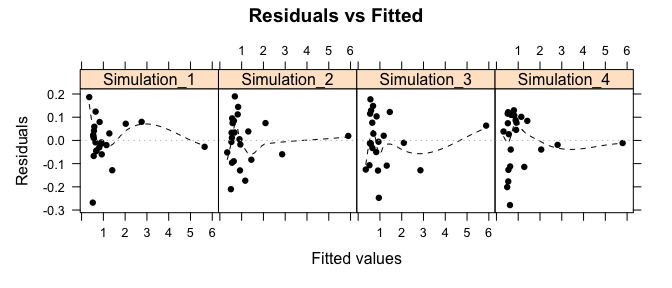Figure 4: Residuals versus fitted values, for four sets of simulated data.

Code is:

plotSimDiags(obj=mftime.lm, which=1, layout=c(4,1))

The simulations indicate that, in these circumstances, there can be a pattern in the smooth curve that is added that is largely due to the one data value is widely separated from other data values.

## A check for normality:

Figure 2 (the second plot) identified two large negative residuals and one large positive residual.

Are the deviations from a line much what might be expected given statistical variation? Figure 5 shows normal probability plots for four sets of simulated data: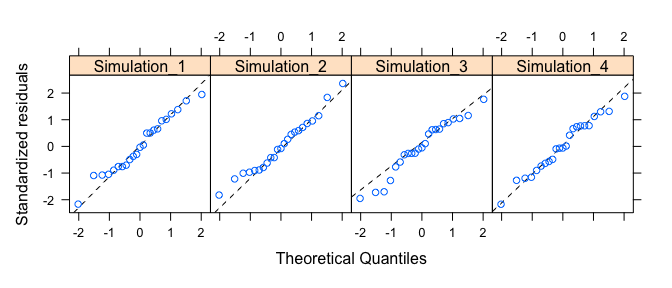Figure 5: Normal probability plots for four sets of simulated data.

Code is as for Figure 4, but with the argument which=2.

## Is the variance constant?

At the low end of the range in Figure 2 (the third plot), the variance hardly changes with increasing fitted value. The sudden bend upwards in the smooth curve is due to the large absolute values of the residuals for the three largest fitted values.

Figure 6 shows the equivalent plots for four sets of simulated data. None of the plots show the same increase in scale with fitted value as in the third panel of Figure 2.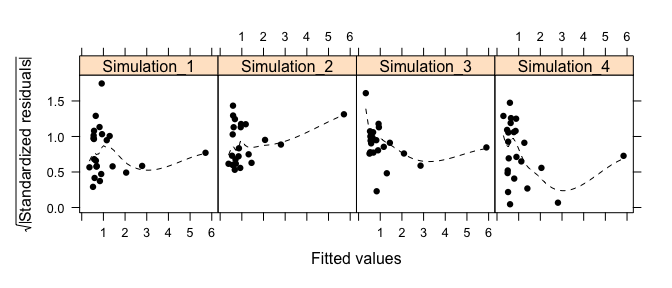Figure 6: Scale-location plots for four sets of simulated data.

Code is as for Figure 4, but with the argument which=3.

## Issues of leverage

Figure 2 (the third plot) warned that there are severe problems with leverage, as was already obvious from the scatterplot in Figure 1. Here, there is not much point in doing a simulation. We already know from the previous simulations that the large residual that is associated with the highest leverage point is unlikely to be due to statistical variation.

Figure 7 shows plots for simulated data: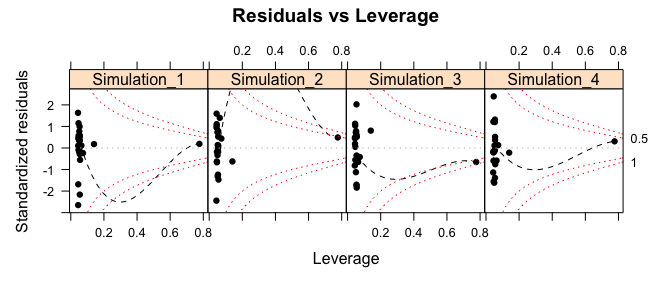Figure 7: Scale-location plots for four sets of simulated data.

Code is as for Figure 4, but with the argument which=5.

## All 4 default diagnostic plots in the same call

Do for example:

gphs1to4 <- plotSimDiags(obj=mftime.lm, layout=c(4,2))

Then gphs1to4[] holds simulations for the first diagnostic plot, gphs1to4[] holds simulations for the second diagnostic plot, and so on.

To show the first set of diagnostic plots, enter:

update(gphs1to4[], layout=c(4,2))

This approach has the advantage that the same simulated data values are used for all diagnostics, without the need to set a prior random number seed.

## What further checks and tests should be applied?

It bears emphasizing that, depending on the nature of the data, there will be further checks and tests that should be applied.

Comments in are apt. There should be incisive and informed critique of the data used, of the model, and of the inferences made. Exposure to diverse challenges will build (or destroy!) confidence in model-based inferences. Specific types of challenge may include:

• For experiments, is the design open to criticism?
• Look for biases in processes that generated the data.
• Look for inadequacies in laboratory procedure.
• Use all relevant graphical or other summary checks to critique the model that underpins the analysis. *Where possible, check the performance of the model on test data that reflects the manner of use of results. (If for example predictions are made that will be applied a year into the future, check how predictions made a year ahead panned out for historical data.)
• For experimental data, have the work replicated independently by another research group, from generation of data through to analysis.

It is important that analysts search out all available information about the processes that generated the data, and consider critically how this may affect the reliance placed on it. We should trust those results that have withstood thorough and informed challenge.

For observational data, the challenges that are appropriate will depend strongly on the nature of the claims made as a result of any analysis. Analyses of observational data commonly afford large opportunities for over-interpretation and/or misinterpretation.

Data that have been collected over a significant period of time is an important special case. Departures from a fitted line may well show a pattern with time. The functions acf() and pacf() should be used to check for autocorrelation in the residuals.

Additionally, the process used to generate the data, and subject area insights, will set limits on the inferences that can reasonably be drawn.